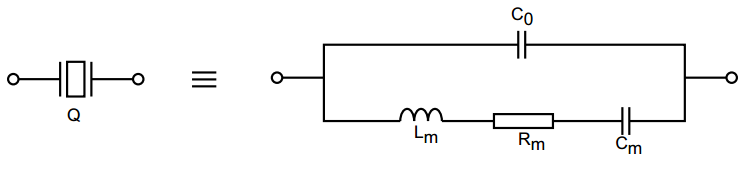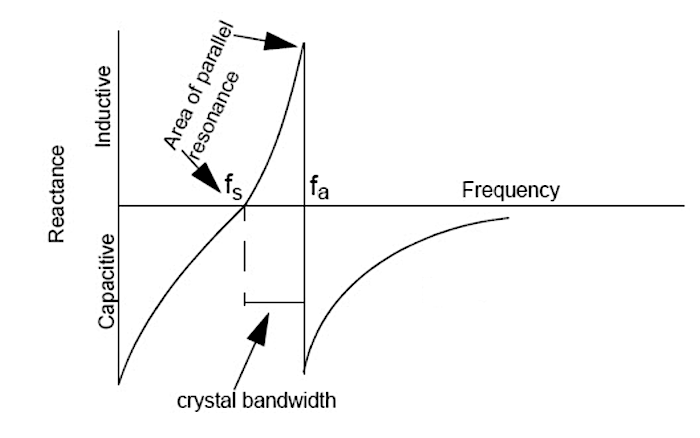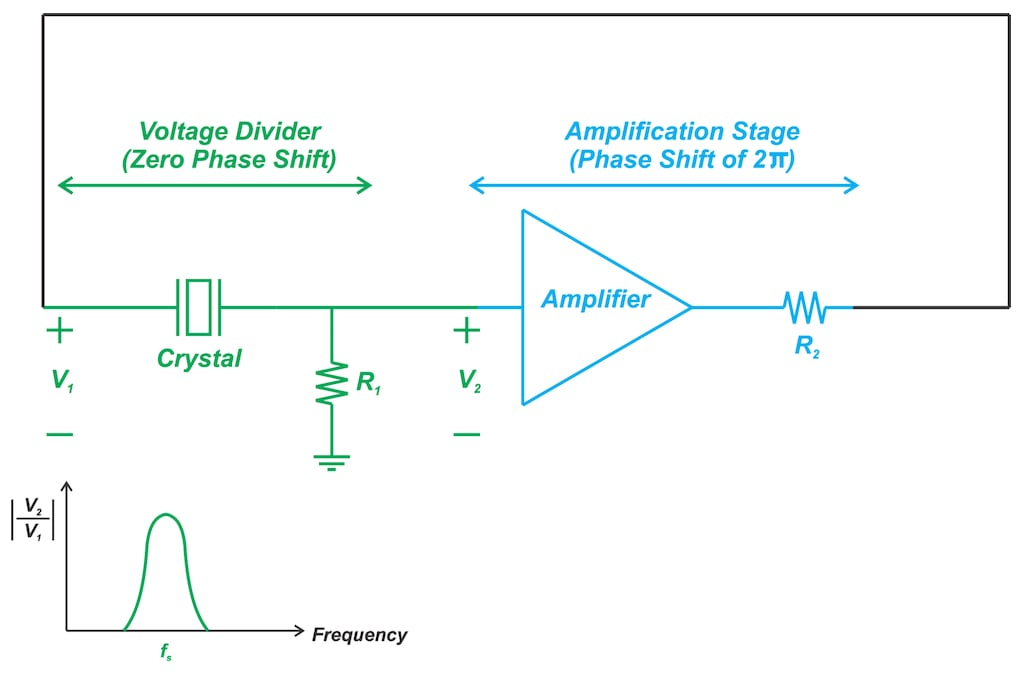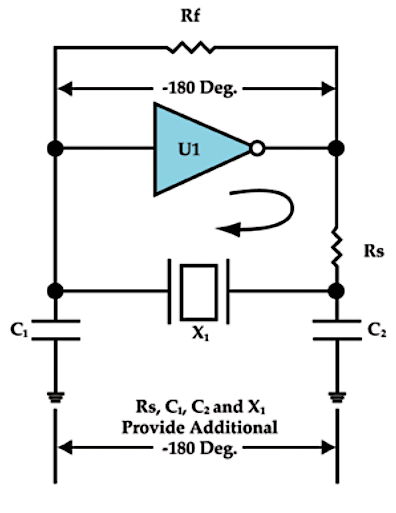Technical Article

# Understanding the Operation of Quartz Crystal Oscillators

June 07, 2021 by Dr. Steve Arar

## In this article, we’ll try to develop a deeper insight into the operation of a quartz crystal oscillator.

In the first part of this series, we looked at some of the important metrics that are used to characterize the frequency deviations of quartz crystals. Now, we'll look more closely at the operation of these devices.

We’ll first examine the reactance vs. frequency curve of a typical crystal. Armed with this knowledge, we’ll take a look at two different oscillator topologies and discuss how the circuit architecture forces the crystal to oscillate at a particular frequency.

Based on this discussion, we’ll be able to look at the definition for parallel and series crystals—two technical terms that can sometimes cause confusion.

### Crystals Have Two Resonant Frequencies

The equivalent electrical circuit for a crystal is shown in Figure 1.##### Figure 1. Image courtesy of STMicroelectronics.

Based on this model, we can find the reactance vs. frequency curve of a typical quartz crystal unit as depicted in Figure 2:##### Figure 2. Image courtesy of Cypress.

To gain some insight into the operation of a crystal, let’s assume that the crystal is ideal and Rm is negligible. Hence, in the lower branch of the crystal electrical model, we have Lm and Cm in series.

When Lm and Cm are in series resonance, their impedances cancel each other out. At this frequency, the impedance of the lower branch and consequently, the total impedance across the crystal drops to zero. This corresponds to fs in Figure 2 that is commonly referred to as the series resonant frequency of the crystal. Note that Co doesn’t affect the value of this frequency.

Just above fs, the reactance of Lm becomes larger than that of Cm and we observe that the crystal exhibits inductive behavior. The reactance of this effective inductance (the series combination of Lm and Cm) increases with frequency and at a certain frequency (fa), it becomes equal to the reactance of Co in the crystal model. At this point, we effectively have a parallel LC in resonance and the total impedance of the crystal approaches infinity. The frequency fa is called the anti-resonant frequency. This frequency is always higher than the series resonant frequency.

### At What Frequency Is the Crystal Going to Oscillate?

We saw that crystals have two modes of resonance. At both fs and fa, the impedance of the crystal is resistive. At fs, the resistance is minimal; however, at the anti-resonant frequency, the equivalent impedance of the crystal approaches infinity.

The question to be asked now is, at what frequency the crystal is going to oscillate when used in an oscillator circuit?

The answer is, it depends on the oscillator topology.

At the oscillation frequency, the loop gain of the oscillator must be equal to or greater than one and its phase shift should be an integral multiple of 2π (positive feedback). These conditions determine the oscillation frequency of the crystal.

As an example, consider the oscillator shown in Figure 3.##### Figure 3

In this case, the phase shift of the amplification stage is an integral multiple of 2π. Hence, at the oscillation frequency, the phase shift caused by the crystal and R1 should be zero. This zero phase shift can be achieved at frequencies that the crystal has a purely resistive impedance (fs and fa).

At fs, the impedance of the crystal is minimal and, hence, the voltage divider created by the crystal and R1 has a larger gain as depicted in the above figure. Therefore, with the above arrangement, the circuit can oscillate at fs.

Another oscillator topology, commonly known as the Pierce-Gate oscillator, is depicted in Figure 4.##### Figure 4. An example Pierce-Gate oscillator. Image courtesy of Ramon Cerda.

With this topology, the amplifier provides a phase shift of 180°. Hence, the network of Rs, C2, C1, and the crystal should provide an additional phase shift of 180° to meet the oscillation phase condition. As the amplifier output signal travels through the feedback path, it experiences some phase shift from the crystal and C1 combination. The amount of this phase shift depends on the signal frequency.

Below fs, the crystal acts as a capacitor, and the phase shift from X1 and C1 is near 0°. At fs, the crystal has resistive impedance and this phase shift is about 90°. Above fs, the crystal exhibits inductive behavior, and the phase shift can be close to 180°.

In practice, the phase shift provided by Rs and C2 is less than 90° and hence, the combination of X1 and C1 needs to provide more than 90°. That’s why the crystal needs to operate somewhere in its inductive region (between fs and fa in Figure 2).

### Parallel-Resonant and Series-Resonant Oscillators

The above discussion shows that a quartz crystal can oscillate at any frequency between the series resonant frequency (fs) and the anti-resonant frequency (fa) depending on the oscillator topology.

Many common oscillator circuits, such as Pierce, Colpitts, and Clapp-style oscillators, operate the crystal in the region between fs and fa. This region is usually called the “area of parallel resonance” and oscillators that force the crystal to operate in this region are called “parallel-resonant oscillators”.

Oscillators that force the crystal to operate at fs are not very common. These oscillators are referred to as “series-resonant oscillators”. It is worthwhile to mention that the anti-resonance point is not used in oscillator designs.

### Parallel and Series Resonant Crystals

There are two technical terms in the crystal industry that can occasionally cause confusion: “parallel-resonant crystal” (or simply parallel crystal) and “series-resonant crystal” (or series crystal).

Parallel crystals are intended for use in parallel-resonant oscillators. Since a parallel-resonant oscillator operates the crystal somewhere between fs and fa, the nominal frequency of a parallel crystal is a frequency in this range, i.e., in the crystal’s “area of parallel resonance”.

On the other hand, a series crystal is intended to be used in series-resonant oscillators. Therefore, the nominal frequency of the crystal is the same as its series resonant frequency (fs).

Is there any physical difference between these two types of crystal?

We know that each crystal has its specific series-resonant frequency and “area of parallel resonance”; and we can operate a given crystal at either of these two resonance conditions. Therefore, there is no difference in the physical structure of parallel and series crystals.

These two terms are only about the conditions under which the crystal will oscillate at its nominal frequency.

Do they specify with what type of oscillator topology the crystal will hit its nominal frequency? Is it a parallel-resonant oscillator or a series-resonant type?